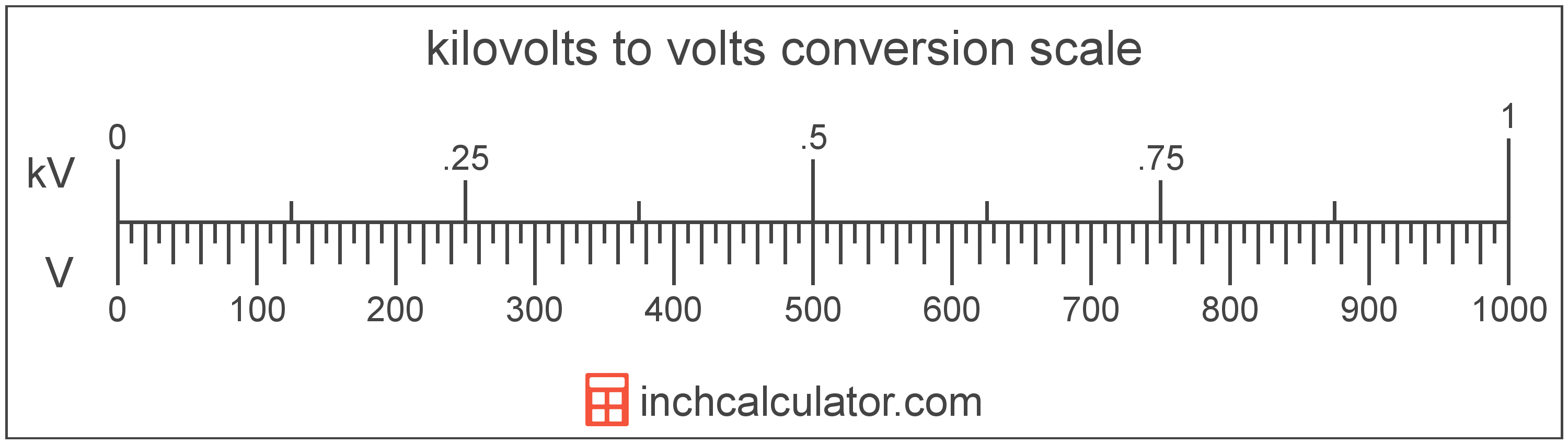# Kilovolts to Volts Converter

Enter the voltage in kilovolts below to get the value converted to volts.

Results in Volts:1 kV = 1,000 V

Do you want to convert volts to kilovolts?

## How to Convert Kilovolts to Volts

To convert a measurement in kilovolts to a measurement in volts, multiply the voltage by the following conversion ratio: 1,000 volts/kilovolt.

Since one kilovolt is equal to 1,000 volts, you can use this simple formula to convert:

volts = kilovolts × 1,000

The voltage in volts is equal to the voltage in kilovolts multiplied by 1,000.

For example, here's how to convert 5 kilovolts to volts using the formula above.
volts = (5 kV × 1,000) = 5,000 V### How Many Volts Are in a Kilovolt?

There are 1,000 volts in a kilovolt, which is why we use this value in the formula above.

1 kV = 1,000 V

## What is a Kilovolt?

One kilovolt is equal to 1,000 volts, which are the potential difference that would move one ampere of current against one ohm of resistance.

The kilovolt is a multiple of the volt, which is the SI derived unit for voltage. In the metric system, "kilo" is the prefix for thousands, or 103. Kilovolts can be abbreviated as kV; for example, 1 kilovolt can be written as 1 kV.

## What is a Volt?

One volt is equal to the potential difference that would move one ampere of current against one ohm of resistance.

The volt is the SI derived unit for voltage in the metric system. Volts can be abbreviated as V; for example, 1 volt can be written as 1 V.

### Voltage and Ohm's Law

Voltage is a measurement of electromotive force and the electrical potential difference between two points of a conductor.

Ohm's Law states the current between two points on a conductor is proportional to the voltage and inversely proportional to the resistance. Using Ohm's Law, it's possible to express the potential difference in volts as an expression using current and resistance.

VV = IA × R

The potential difference in volts is equal to the current in amperes times the resistance in ohms.

## Kilovolt to Volt Conversion Table

Table showing various kilovolt measurements converted to volts.
Kilovolts Volts
0.001 kV 1 V
0.002 kV 2 V
0.003 kV 3 V
0.004 kV 4 V
0.005 kV 5 V
0.006 kV 6 V
0.007 kV 7 V
0.008 kV 8 V
0.009 kV 9 V
0.01 kV 10 V
0.02 kV 20 V
0.03 kV 30 V
0.04 kV 40 V
0.05 kV 50 V
0.06 kV 60 V
0.07 kV 70 V
0.08 kV 80 V
0.09 kV 90 V
0.1 kV 100 V
0.2 kV 200 V
0.3 kV 300 V
0.4 kV 400 V
0.5 kV 500 V
0.6 kV 600 V
0.7 kV 700 V
0.8 kV 800 V
0.9 kV 900 V
1 kV 1,000 V

## References

1. International Bureau of Weights and Measures, The International System of Units, 9th Edition, 2019, https://www.bipm.org/documents/20126/41483022/SI-Brochure-9-EN.pdf